﻿ 直流接地极土壤电阻率建模方法研究

# 直流接地极土壤电阻率建模方法研究Research on Soil Resistivity Modeling Method of DC Grounding Electrode

Abstract: The DC ground electrode is an important part of the DC transmission project. The distribution of the soil resistivity around it directly affects the parameter design and operation and maintenance of the ground electrode. In order to ensure the normal operation of the ground electrode and avoid af-fecting the personal safety and equipment performance near the ground electrode, it is necessary to test and model the soil resistivity around the ground electrode. In this paper, the four-pole method and the magnetotelluric method are used to test the soil resistivity around the ground electrode. The results obtained by these two test methods are compared, and the respective advantages and disadvantages of these two methods in soil resistivity testing are comprehensively analyzed. The soil resistivity is modeled by the inversion software independently developed by our team. Finally, the grounding resistance value calculated according to the soil model is in good agreement with the reference value, which verifies the reliability and accuracy of the method.

1. 引言

2. 四极电法和大地电磁法的测试原理

2.1. 四极电法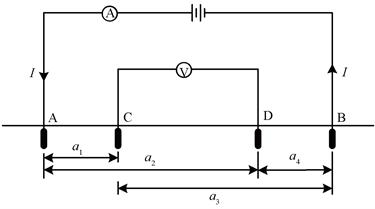Figure 1. Schematic diagram of soil resistivity measurement

${V}_{A}=\frac{I\rho }{2\pi x}$ (1)

${V}_{B}=-\frac{I\rho }{2\pi x}$ (2)

${V}_{B}=\frac{I\rho }{2\pi }\left(\frac{1}{{a}_{1}}-\frac{1}{{a}_{2}}\right)$ (3)

${V}_{D}=\frac{I\rho }{2\pi }\left(\frac{1}{{a}_{2}}-\frac{1}{{a}_{4}}\right)$ (4)

${U}_{CD}=\frac{I\rho }{2\pi }\left(\frac{1}{{a}_{1}}-\frac{1}{{a}_{2}}-\frac{1}{{a}_{3}}+\frac{1}{{a}_{4}}\right)$ (5)

$\rho =\frac{2\pi R}{\frac{1}{{a}_{1}}-\frac{1}{{a}_{2}}-\frac{1}{{a}_{3}}+\frac{1}{{a}_{4}}}$ (6)

$R=\frac{{U}_{CD}}{I}$ (7)

2.2. 大地电磁法

$p=\frac{1}{2\pi }\sqrt{\frac{10\rho }{f}}\approx 503\sqrt{\frac{\rho }{f}}$ (8)

3. 土壤电阻率现场测试

3.1. 现场选址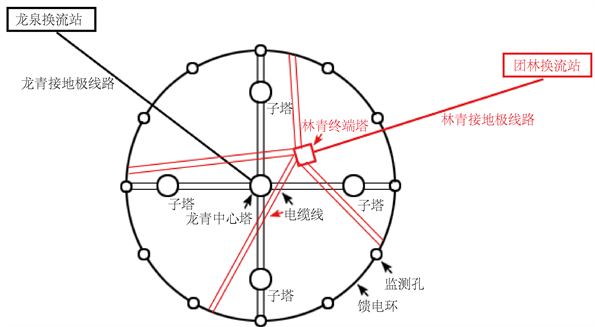Figure 2. Longqing ground electrode and Linqing ground electrode share the Qingtai pole site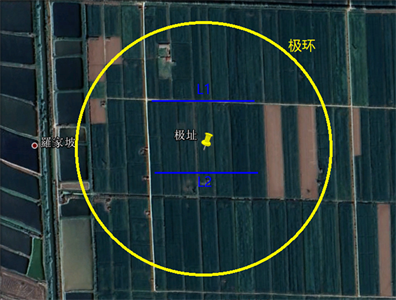Figure 3. L1, L2 measuring line position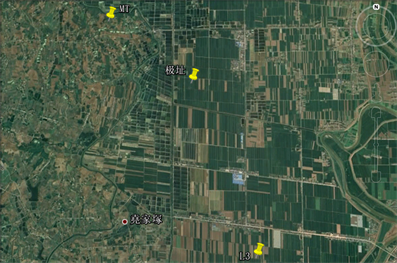Figure 4. L3 survey line, MT survey point location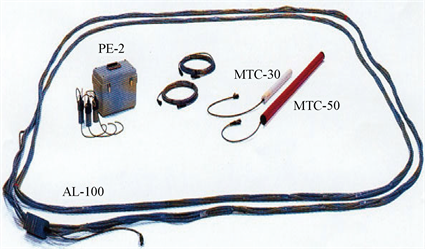Figure 5. V5-2000 system magnetic sensors and electrodes

3.2. 测试结果及反演

3.2.1. 四级法土壤电阻率测试结果与反演Table 1. Measurement results of L1 and L2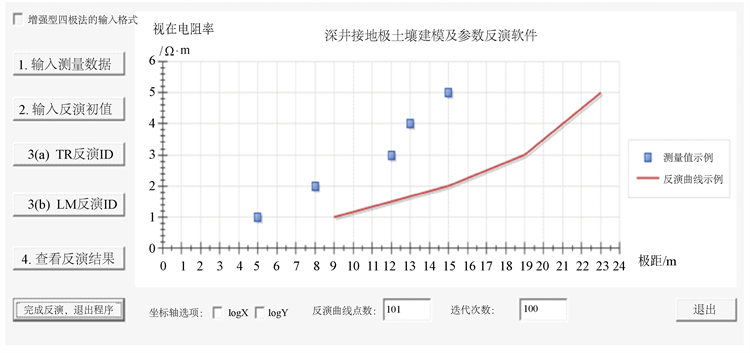Figure 6. The main interface of the soil inversion software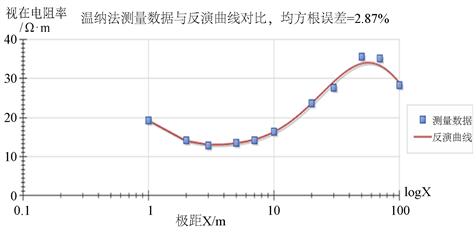Figure 7. Inversion results of L1 survey line

Table 3. Inversion of soil layering model by L1 survey lineTable 4. L2 line inversion soil layering modelTable 5. L3 line inversion soil layering model

3.2.2. 大地电磁法MT土壤电阻率测试结果Table 6. MT inversion soil layering model

4. 土壤电阻率综合分析与验证

4.1. 土壤电阻率测试方法的分析比较Table 7. Recommended soil resistivity

4.2. 土壤电阻率测试结果的验证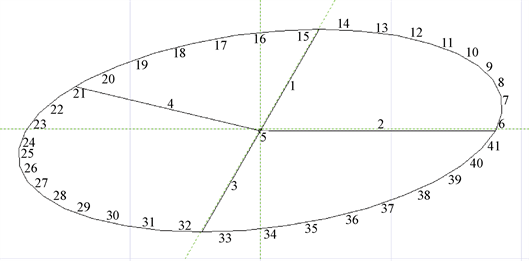Figure 8. Simulation model of DC ground electrode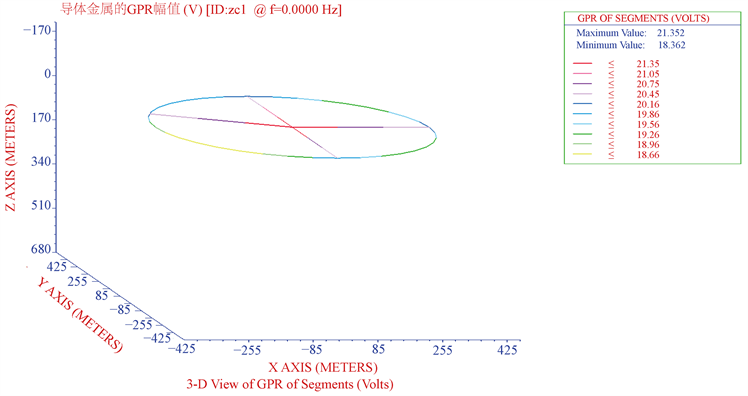Figure 9. Electric potential distribution on the surface of the ground conductor

4.3. 小结

5. 结论

1) 由于龙泉、团林共用青台接地极址位于耕地区域，接地极附近土壤的电阻率普遍较低。大部分区域的土壤电阻率低于30 Ω∙m，电阻率最高区域的土壤电阻率为66.9 Ω∙m；

2) 在120 m范围内，四极法测量土壤电阻率的精度较高；在120 m以下时，土壤电阻率突然增大，反演结果均方根误差过大。表明四极法对浅层土壤电阻率测试的精度较高，随着测试深度的增加，大地电磁法的优势越来越明显；

3) 利用反演得到的土壤模型，仿真计算得到接地极的接地电阻值为0.21 Ω，与该接地极前期测试得到的接地电阻值为0.028~0.029 Ω吻合较好，进一步验证了本文提出的土壤电阻率建模方法的有效性和可靠性。

 刘连光, 崔明德, 孙中明, 等. ±800 kV直流接地极对交流电网的影响范围[J]. 高电压技术, 2009, 35(6): 1243-1247.

 胡毅. 直流接地极电流对输电线路接地构件的腐蚀影响研究[J]. 中国电力, 2000, 3(1): 59-61.

 曾连生. 直流输电接地极电流对电力变压器的影响[J]. 高电压技术, 2005, 31(4): 57-58.

 马成廉, 孙黎, 尚教会. 抗灾型电网安全风险评估方法研究[J]. 电网与清洁能源, 2014, 30(9): 12-18.

 赵婉君. 高压直流输电工程技术[M]. 第2版. 北京: 中国电力出版社, 2011.

 李景丽, 冯鹏, 栗超超. 深井型直流接地极散流机理研究[J]. 电瓷避雷器, 2020(4): 87-94.

 陈坤汉, 杨道武, 朱志平, 杨海军. 接地网在土壤中的腐蚀特性研究[J]. 电瓷避雷器, 2008(4): 39-42 + 46.

 Nayel, M., Lu, B., Tian, Y., et al. (2012) Study of Soil Resistivity Measurements in Vertical Two-Layer Soil Model.

 颜喜平, 许根养, 敬亮兵, 张杰, 江龙才, 李志娟, 马福, 李景禄. 现场杆塔接地电阻和土壤电阻率测量存在问题及误差分析[J]. 电瓷避雷器, 2008(3): 38-41.

 苏杰, 吴广宁, 曹晓斌, 等. 应用可控源音频大地电磁法的土壤电阻率测量[J]. 电网技术, 2011, 35(1): 141-145.

 朱德朋. 磁法与大地电磁法数据二维联合反演研究[D]: [硕士学位论文]. 北京: 中国地质大学, 2015.

 马成廉, 刘利则, 徐冰, 等. 特高压直流输电接地极大地电阻率测量方法研究[J]. 电网与清洁能源, 2015, 31(3): 6-10, 17.

 解广润. 电力系统接地技术[M]. 北京: 中国电力出版社, 1996.

 杨生. 大地电磁测深法环境噪声抑制研究及其应用[D]: [博士学位论文]. 长沙: 中南大学, 2004.

 杨磊, 张志勇, 李曼, 等. 直流电阻率法与大地电磁法的二维联合反演[J]. 地球物理学进展, 2016, 31(2): 851-855.

 丁文伟. 直流电阻率法与音频大地电磁法二维联合反演研究[D]: [硕士学位论文]. 抚州: 东华理工大学, 2019.

 袁志军, 王峥. ±500 kV龙泉换流站接地极运行分析[J]. 华北电力技术, 2006(10): 52-54.

Top